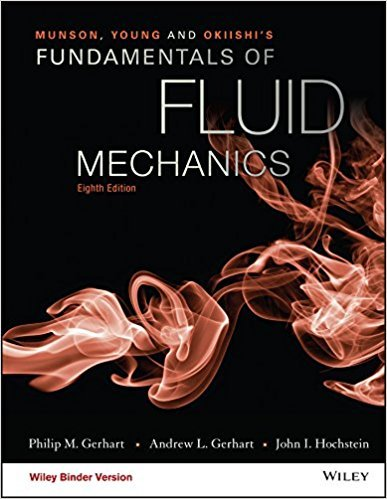×
Get Full Access to Fundamentals Of Fluid Mechanics - 8 Edition - Chapter 7.6 - Problem 7.36
Get Full Access to Fundamentals Of Fluid Mechanics - 8 Edition - Chapter 7.6 - Problem 7.36

×

# The following dimensionless groups are often used to presentdata on centrifugal pumpsISBN: 9781119080701 456

## Solution for problem 7.36 Chapter 7.6

Fundamentals of Fluid Mechanics | 8th Edition

• Textbook Solutions
• 2901 Step-by-step solutions solved by professors and subject experts
• Get 24/7 help from StudySoup virtual teaching assistantsFundamentals of Fluid Mechanics | 8th Edition

4 5 1 396 Reviews
18
1
Problem 7.36

The following dimensionless groups are often used to presentdata on centrifugal pumps: flow coefficient = QD3, headcoefficient = gH2D2, power coefficient = W3D5, efficiency =gQHW , specific speed Ns = Q(gh)3/4, specific diameter Ds = Q(gh)1/4.Show that the last three groups can be formed from combinationsof the first three groups.

Step-by-Step Solution:
Step 1 of 3

Cyclones and Anticlones • Cyclone o A center of low pressure o Pressure decreased toward the center o Winds associated with cyclone ▪ Northern hemisphere • Inward convergence • Counterclockwise ▪ Southern hemisphere • Inward convergence • Clockwise • Associated with rising air • Anticyclone o A center of high pressure o Pressure increases toward the center o Wind associated with an anticyclone ▪ Northern hemisphere • Outward divergence • Clockwise ▪ Southern hemisphere • Outward divergence • Clockwise • General atmospheric circulation o Underlying cause is unequal surface heating o On the rotating earth 3 pairs of atmospheric cells the redistributed heat o Idealized global circulation ▪ Equatorial low-pressure zone ▪ Subtropical high-pressure zone ▪ Trade winds ▪ Westerly winds ▪ Subpolar low-pressure zone ▪ Polar high ▪ Polar easterly/westerly o Influence of continents ▪ Seasonal temp differences disrupt • Global pressure patterns • Global wind pattern o Influence must originate in North Hemisphere ▪ Monsoon • Seasonal change • Circulation in the mid-latitudes o The zone of the westerlies o Complex o Air flow is interrupted by cyclones • Local wind

Step 2 of 3

Step 3 of 3

##### ISBN: 9781119080701

Fundamentals of Fluid Mechanics was written by and is associated to the ISBN: 9781119080701. The full step-by-step solution to problem: 7.36 from chapter: 7.6 was answered by , our top Science solution expert on 03/16/18, 03:21PM. The answer to “The following dimensionless groups are often used to presentdata on centrifugal pumps: flow coefficient = QD3, headcoefficient = gH2D2, power coefficient = W3D5, efficiency =gQHW , specific speed Ns = Q(gh)3/4, specific diameter Ds = Q(gh)1/4.Show that the last three groups can be formed from combinationsof the first three groups.” is broken down into a number of easy to follow steps, and 50 words. This textbook survival guide was created for the textbook: Fundamentals of Fluid Mechanics, edition: 8. Since the solution to 7.36 from 7.6 chapter was answered, more than 255 students have viewed the full step-by-step answer. This full solution covers the following key subjects: . This expansive textbook survival guide covers 112 chapters, and 1357 solutions.

## Discover and learn what students are asking

Statistics: Informed Decisions Using Data : An Overview of Nonparametric Statistics
?List the disadvantages of using nonparametric statistical procedures

Statistics: Informed Decisions Using Data : Inference about Measures of Central Tendency
?Write a paragraph that describes the logic of the test statistic in a two-tailed sign test.

Statistics: Informed Decisions Using Data : Inference about the Difference between Two Medians: Dependent Samples
?In Problems 3–10, use the Wilcoxon matched-pairs signedranks test to test the given hypotheses at the a = 0.05 level of significance. The dependent sa

Unlock Textbook Solution